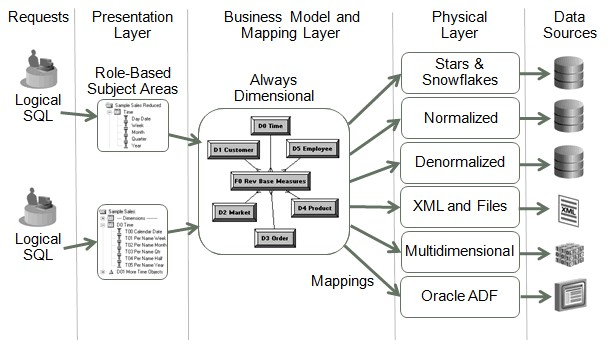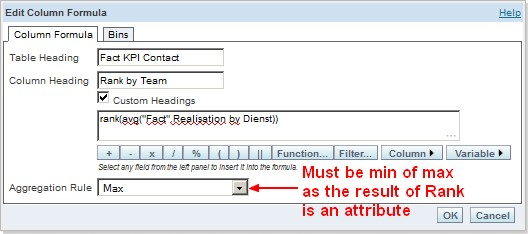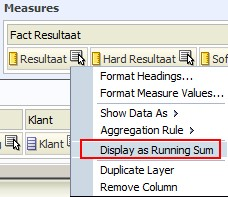# OBIEE - (Display Function|Derived Measures) (Rank, TopN, Median, )

Oracle BI Answers simplifies the use of derived measures i.e. measures that are computed on a query result set such as ranks, Ntiles, standard deviations, running totals, moving averages, and moving medians.

A display function (or derived measure) is a (function|measure) that (operates|computes) on a on the result set of a query.

The display functions the Analytics Server supports are:

• Rank,
• TOPn,
• BOTTOMn,
• PERCENTILE,
• NTILE,
• MAVG,
• and varieties of standard deviation

are specified in the SELECT list of a SQL query.

These derived measures are difficult to compute in SQL but are very useful — moving average and moving median are valuable functions for smoothing data and discerning trends.

## Aggregate Rule

Queries that use display functions conform to the following rules:

• If no GROUP BY clause is specified, the display function operates across the entire result set; that is, the grouping level for the display function is the same as for the query.
• If there is a GROUP BY clause specified, the display function resets its values for each group as specified in the GROUP BY clause.

For example, consider the following query, where SumOfRevenue is defined as a measure column with the default aggregation rule of SUM:

``````select year, product, SumOfRevenue, rank(SumOfRevenue)
from time, products, facts```
```
``````YEAR    PRODUCT     SUMOFREVENUE RANK(SUMOFREVENUE)
1998    Coke         500          6
1998    Pepsi        600          2
1999    Coke         600          2
1999    Pepsi        550          5
2000    Coke         800          1
2000    Pepsi        600          2
```
```

In this query result set, there is no GROUP BY clause specified, so the rank is calculated across the entire result set. The query is logically the same query as the following:

select year, product, SumOfRevenue, rank(SumOfRevenue)) from time, products, facts group by year, product

If you change the GROUP BY clause to only group by year, then the rank is reset for each year, as follows:

``````select year, product, sum(revenue), rank(sum(revenue))
from time, products, facts
group by year```
```
``````YEAR    PRODUCT     SUM(REVENUE) RANK(SUM(REVENUE))
1998    Coke         500          2
1998    Pepsi        600          1
1999    Coke         600          1
1999    Pepsi        550          2
2000    Coke         800          1
2000    Pepsi        600          2
```
```

In this result set, the rank is reset each time the year changes, and because there are two rows for each year, the value of the rank is always either 1 or 2.

## Reference

Recommended PagesOBIEE - Functions

The home page of OBIEE functions of the logical sql and from BI Server. See Logical SQL ReferenceOBIEE - Rank

Rank function in OBIEE. This function in OBIEE follows the same principles that all display functions.OBIEE - Running Sum (RSum)

Running Sum is a display function. This function calculates a running sum based on records encountered so far. The “By” statement resets its values for each group. Logical SQL: Result: ...